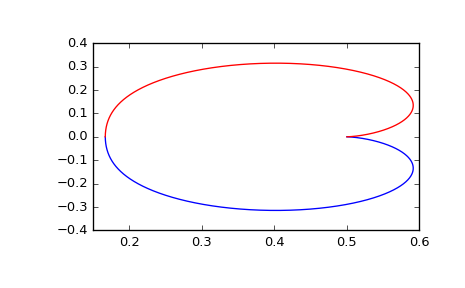# scipy.signal.dfreqresp¶

scipy.signal.dfreqresp(system, w=None, n=10000, whole=False)[source]

Calculate the frequency response of a discrete-time system.

Parameters: system : an instance of the dlti class or a tuple describing the system. The following gives the number of elements in the tuple and the interpretation: 1 (instance of dlti) 2 (numerator, denominator, dt) 3 (zeros, poles, gain, dt) 4 (A, B, C, D, dt) w : array_like, optional Array of frequencies (in radians/sample). Magnitude and phase data is calculated for every value in this array. If not given a reasonable set will be calculated. n : int, optional Number of frequency points to compute if w is not given. The n frequencies are logarithmically spaced in an interval chosen to include the influence of the poles and zeros of the system. whole : bool, optional Normally, if ‘w’ is not given, frequencies are computed from 0 to the Nyquist frequency, pi radians/sample (upper-half of unit-circle). If whole is True, compute frequencies from 0 to 2*pi radians/sample. w : 1D ndarray Frequency array [radians/sample] H : 1D ndarray Array of complex magnitude values

Notes

If (num, den) is passed in for system, coefficients for both the numerator and denominator should be specified in descending exponent order (e.g. z^2 + 3z + 5 would be represented as [1, 3, 5]).

New in version 0.18.0.

Examples

Generating the Nyquist plot of a transfer function

>>> from scipy import signal
>>> import matplotlib.pyplot as plt


Transfer function: H(z) = 1 / (z^2 + 2z + 3)

>>> sys = signal.TransferFunction(, [1, 2, 3], dt=0.05)

>>> w, H = signal.dfreqresp(sys)

>>> plt.figure()
>>> plt.plot(H.real, H.imag, "b")
>>> plt.plot(H.real, -H.imag, "r")
>>> plt.show()#### Previous topic

scipy.signal.dstep

#### Next topic

scipy.signal.dbode